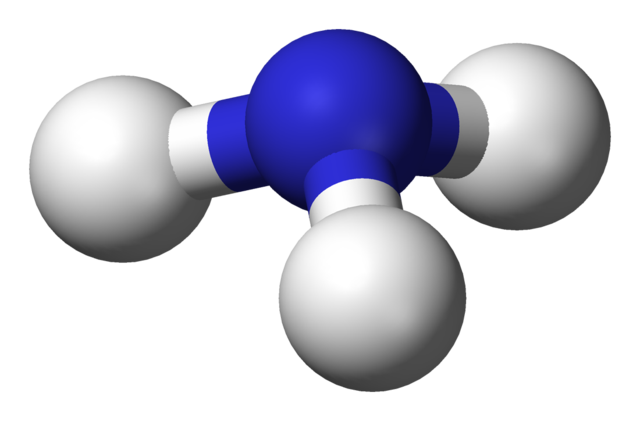10 minutes maximum! Can you do it in 5?

1. The equation to calculate percentage yield is ...
• A.   percentage yield = (actual mass of product/maximum theoretical mass of product) x 100
• B.   percentage yield = (maximum theoretical mass of product/actual mass of product) x 100
• C.   percentage yield = (actual mass of product/mass of reactant used) x 100
• D.   percentage yield = (mass of reactant used/actual mass of product) x 100

2. Tungsten metal was produced by reducing tungsten fluoride with hydrogen. A student calculated that a mass of 52.0g of tungsten should be formed. He actually obtained 43.2g of tungsten.
What is the percentage yield of tungsten in this experiment?

• A. 16.9%
• B. 83.0%
• C. 83.1%
• D. 120%

Q3+4: A student synthesized 12.3g of aspirin from 13.8g of salicylic acid. He calculated that the maximum possible mass of aspirin which he could obtain was 18.0g.3. a possible reason for the student obtaining less aspirin than expected is ...

• A. the reaction may not have gone to completion
• B. some aspirin may have been lost during separation from the reaction mixture
• C. some of the salicylic acid may have reacted to produce another product
• D. all of the above

4. The percentage yield of aspirin in this experiment was:

• A. 112%
• B. 89.1%
• C. 76.7%
• D. 68.3%

Q5+6.

21.7g of mercury(II)oxide was decomposed by heating and 9.0g of mercury was collected.

The equation for the reaction is:

2HgO (s)      2Hg (l)   +  O2 (g)

5. The mass of 1 mole of mercury(II)oxide and the number of moles in 21.7g is:

 Mass of 1 mole of mercury(II)oxide  /g Number of moles in 21.7g A 217 0.1 B 217 0.05 C 88 0.25 D 88 0.12

6. The maximum mass of mercury which could be obtained in this experiment and the % yield are:

 Maximum possible mass of mercury  /g % yield A 10.1 89.1 B 10.1 112.2 C 20.1 44.8 D 20.1 223.3

Q7+8.

50g of calcium carbonate was strongly heated resulting in thermal decomposition.
The equation for the reaction is:

CaCO3 (s  CaO (s) +   CO2 (g)7. What is the molar mass of calcium carbonate and how many moles of CaCO3 are in 50 g?

 Molar mass of CaCO3 /g Number of moles of CaCO3 in 50g A 68 1.36 B 68 0.74 C 100 2 D 100 0.5
8. What mass of calcium oxide is obtained from the thermal decomposition of 50 g of calcium carbonate if the reaction has a 40% yield?
• A.   11.2g
• B.   16.6g
• C.   16.8g
• D.   28.0g

Q9+10.
A manufacturer produced 51kg of ammonia by reacting 56kg of nitrogen with excess hydrogen in the Haber process.
The equation for this reaction is:

N2 + 3H2 ⇌ 2NH39. The maximum possible yield of ammonia, if the reaction went to completion, is:

• A. 34kg
• B. 56kg
• C. 68kg
• D. 136kg

10. The percentage yield of ammonia in this reaction is:

• A. 38%
• B. 75%
• C. 82%
• D. 91%